﻿ A New Collocation Method for Systems of Nonlinear Fredholm Integral Equations

### A New Collocation Method for Systems of Nonlinear Fredholm Integral Equations

S.A. Edalatpanah, E. AbdolmalekiOPEN ACCESSPEER-REVIEWED

## A New Collocation Method for Systems of Nonlinear Fredholm Integral Equations

S.A. Edalatpanah1,, E. Abdolmaleki1

1Department of Mathematics, Tonekabon Branch, Islamic Azad University, Tonekabon, Iran

### Abstract

In this paper we present a new method for solving nonlinear Fredholm integral equations system in terms of continuous Legendre multi-wavelets on the interval [0, 1). To begin with we describe the characteristic of Legendre multi-wavelets and will go on to indicate that through this method a system of Fredholm integral equations can be reduced to an algebraic equation. Convergence analysis of this method is also presented. Finally, numerical results are given which support the theoretical results.

• Edalatpanah, S.A., and E. Abdolmaleki. "A New Collocation Method for Systems of Nonlinear Fredholm Integral Equations." Applied Mathematics and Physics 2.1 (2014): 15-18.
• Edalatpanah, S. , & Abdolmaleki, E. (2014). A New Collocation Method for Systems of Nonlinear Fredholm Integral Equations. Applied Mathematics and Physics, 2(1), 15-18.
• Edalatpanah, S.A., and E. Abdolmaleki. "A New Collocation Method for Systems of Nonlinear Fredholm Integral Equations." Applied Mathematics and Physics 2, no. 1 (2014): 15-18.

 Import into BibTeX Import into EndNote Import into RefMan Import into RefWorks

### 1. Introduction

The subject of integral equations is one of the most important mathematical tools in both pure and applied mathematics. Integral equations play a very important role in modern science such as numerous problems in engineering and mechanics, for more details see [1, 2, 3]. For such equations as well as a system of such equations, various techniques such as iterative, extrapolation, Galerkin, collocation, quadrature, projection, spline, orthogonal polynomial, and multiple grid methods have been presented to determine desired solutions (see e.g. [1, 2, 3] and the references quoted there).

Let us consider the system of non linear Fredholm integral equations of the form: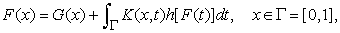(1)

where,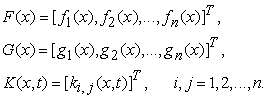In system (1) the known kernel K (x, t) is continuous, the function G (x) is given, and F (x) is the solution to be determined .

There have been considerable interests in solving integral equation (1). In addition to the well-known techniques, there are several new techniques for solving integral equation systems [5-17]. As we know, it is important to select a suitable basis function in numerical methods for system of integral equations. One of the most attractive proposals made in the recent years was an idea connected to the application of wavelets as basis functions in the method of moments . The wavelet technique allows the creation of very fast algorithms when compared to the algorithms ordinarily used and the main advantage of the wavelet technique is its ability to transform complex problems into a system of algebraic equations. Various wavelet basis are applied. In addition to the conventional Duabechies wavelets, Haar wavelets , linear B-splines , Walsh functions  have been used.

In this paper, we propose the application of the linear Legendre multi-wavelets as basis functions in collocation’s method for numerical solution of the system of nonlinear Fredholm integral equations (1). The method is tested with the numerical examples.

### 2. Legendre Multi-Wavelets

Wavelets constitute a family of functions constructed from dilation and translation of a single function called the mother wavelet. When the dilation parameter a and the translation parameter b vary continuously, we have the following family of continuous wavelets as .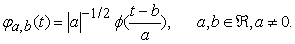If we restrict the parameters a and b to discrete values as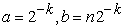, then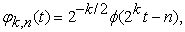form an orthogonal basis .

The linear Legendre multi-wavelets are described in  and applied in [23, 24, 25, 26]. For constructing the linear Legendre multi-wavelets, at first we describe the following scaling functions;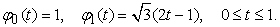Now let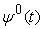and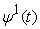be the corresponding mother wavelets, then by Multiresolution of analysis (MRA) and applying suitable conditions  onandthe explicit formula for linear Legendre mother wavelets will obtain as;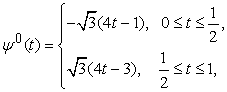(2)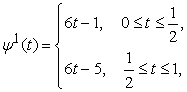(3)

and the family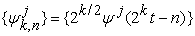, k is any nonnegative integer,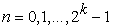and j = 0, 1, forms an orthonormal basis for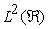.

### 3. Function Approximation

A function f (t) defined over [0, 1) can be expanded as;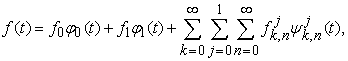(4)

where,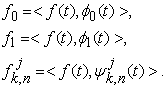(5)

In Eq.(5),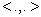denoting the inner product. If the infinite series of Eq.(4) is truncated, then it can be written as;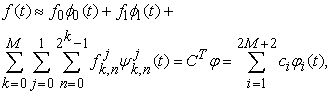(6)

where,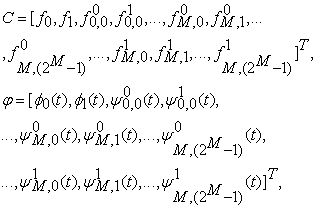and M is a nonnegative integer.

### 4. System of Nonlinear Fredholm Integral Equations

In this section we apply collocation method to convert Eq.(1) to algebraic system of nonlinear equations and then solve this system by well known solver. We assume that Eq. (1) has a unique solution. However, the necessary and sufficient conditions for existence and uniqueness of the solution of system Eq.(1) could be found in . We approximate fi (x)’s, such that;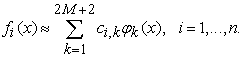(7)

where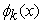defined in Eq. (6) and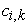’s are unknown coefficients which are determined by solving algebraic system.

By substituting Eq. (7) in Eq. (1) we have;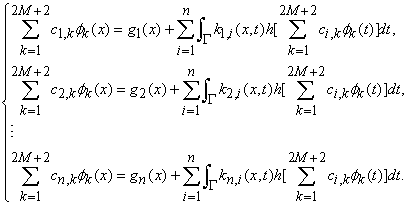Now, we choose some collocation points such as;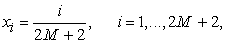which are equidistant, also define system of residual equations by: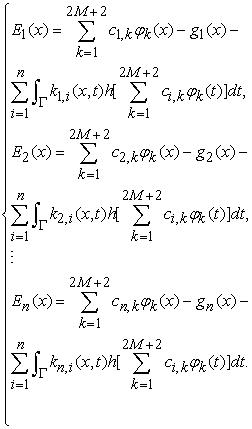Then, by imposing the conditions;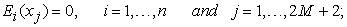we can conclude algebraic system of nonlinear equations.

Next, we present the convergence analysis of our method.

Let us consider the following norms;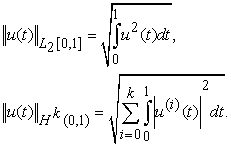Lemma 4.1. Let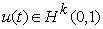(Sobolev space) and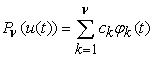be the best approximation polynomial of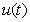in L2-norm. Then we have,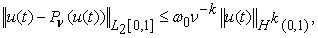where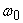is a positive constant, which depends on the selected norm and is independent ofand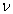.

Proof. .

Theorem 4.1. Le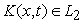and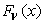be the numerical solution of the Eq. (1). Then we have;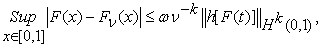where,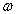is a positive constant.

Proof. By Eq.(1), we get;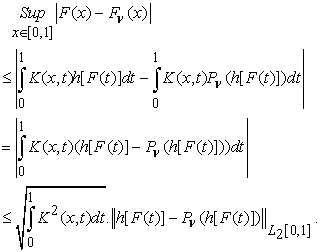Since,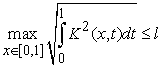Furthermore, by Lemma 4.1, we obtain;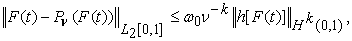and therefore,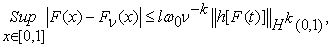and by choosing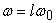the proof is completed.

### 5. Numerical Experiments

In this section, we give some numerical experiments to illustrate the results obtained in previous sections. All the numerical experiments presented in this section were computed by a Maple 16 on a PC with a 1.86GHz 32-bit processor and 1GB memory. Moreover, these examples are solved by M = 1.

Example 5.1. Consider the following nonlinear system of Fredholm integral equations: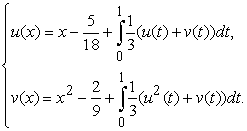With the exact solutions u (x) = x and v (x) = x2.

Table 1 is the numerical results for Example 5.1.

#### Table 1. Shows the results of example 5.1

Example 5.2. Consider the following nonlinear system of Fredholm integral equations: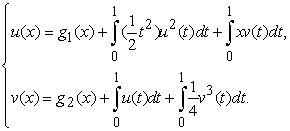Where;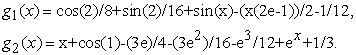With the exact solutions u (x) = sin(x) and v (x) = x+ex.

Table 2 is the numerical results for Example 5.2.

### 6. Conclusions

In this paper, the systems of nonlinear Fredholm integral equations are investigated and a practical projection method known as collocation method based on Legendre multi-wavelets is established. Finally, illustrative examples are included to demonstrate the validity and applicability of the technique.

### References

  K.E. Atkinson, The numerical solution of integral equations of the second kind, Cambridge University Press, Cambridge, 1997.In article CrossRef  R. Kress, Linear integral equations, Springer-Verlag, NewYork, 1999.In article  P.K. Kythe, P. Puri, Computational methods for linear integral equations, BirkhauserVerlag, Springer, Boston, 2002.In article CrossRef  L.M. Delves, J.L. Mohamed, Computational methods for integral equations, Cambridge University Press, London, 1985.In article CrossRef  K. Maleknejad, F. Mirzaee, Numerical solution of linear Fredholm integral equations system by rationalized Haar functions method. Int J Comput Math 80 (2003) 1397-405.In article CrossRef  E. Babolian, J. Biazar, and A.R. Vahidi, The decomposition method applied to system of Fredholm integral equations of the second kind, Appl. Math. Comput. 148 (2004) 443-452.In article CrossRef  K. Maleknejad, M. Sharezaee, and H. Khatami, Numerical solution of integral equations system of the second kind by Block-Pulse functions, Appl. Math. Comput. 166 (2005) 15-24.In article CrossRef  K. Maleknejad, M. Shahrezaee, Rung-Kutta method for numerical solution of the system of Volterra integral equation, Appl. Math. Comput., 149 (2004) 399-410.In article CrossRef  J. Pour-Mahamoud, M.Y. Rahimi-Ardabili, and S. Shahmorad, Numerical solution of system of Fredholm integro-differential equations by the Tau method, Appl. Math. Comput. 168 (2005) 465-478.In article CrossRef  K. Ivaz, B.S.Mostahkam, Newton-Tau numerical solution of a system of nonlinear Fredholm integral equations of second kind. Appl. Comput. Math., 5 (2006) 201-208.In article  M. Gulsu and M. Sezer, Taylor collocation method for solution of systems of high-order linear Fredholm–Volterra integro-differential equations, Int. J. Comput. Math. 83 (2006) 429-448.In article CrossRef  J. Rashidinia, M. Zarebnia, Convergence of approximate solution of system of Fredholm integral equations. J Math Anal Appl, 333 (2007) 1216-27.In article CrossRef  R.K. Saeed, Homotopy perturbation method for solving system of nonlinear Fredholm integral equations of the second kind. Journal of Applied sciences Research, 10 (2008) 1166-1173.In article  M.I.Berenguer, G. Gamez, A.I. Garralda-Guillem, M.Ruiz Galan, M.C. Serrano Perez, Biorthogonal systems for solving Volterra integral equation systems of the second kind. J. Comput. Appl.Math. 235 (2011) 1873-1875.In article CrossRef  H. Almasieh, M. Roodaki, Triangular functions method for the solution of Fredholm integral equations system, Ain Shams Engineering Journal., 3 (2012) 411-416.In article CrossRef  S. Ding, H Yang, Fast multiscale Galerkin methods for solving ill-posed integral equations via a coupled system under general source conditions, J. Math. Anal. Appl. (2013).In article CrossRef  W. Jiang, Z. Chen, Solving a system of linear Volterra integral equations using the new reproducing kernel method, Applied Mathematics and Computation. 219 (2013) 10225-10230.In article CrossRef  A.P. Anioutine, A.G. Kyurkchan, Application of wavelets technique to the integral equations of the method of auxiliary currents,J. Quant. Spectrosc. Radiat. Transf. 79-80 (2003) 495-508.In article CrossRef  B.A. Lewis, On the numerical solution of Fredholm integral equations of the ﬁrst kind, J. Inst. Math. Appl. 16 (1973) 207-220.In article CrossRef  C. Sanchez-Avila, Wavelet domain signal deconvolution with singularity-preserving regularization, Math. Comput. Simul. 61 (2003) 165-176.In article CrossRef  A. Boggess, F. J. Narcowich, A First Course in Wavelets with Fourier Analysis, Prentice-Hall, 2001.In article  F. Khellat, S. A. Youseﬁ, The linear mother wavelets operational matrix of integration and its application, Journal of Franklin Institute, 343 (2006), 181-190.In article CrossRef  H. Danfu, S. Xufeng, Numerical solution of Fredholm integral equations of the first kind by using linear Legendre multi-wavelets, Applied Mathematics and Computation., 191 (2007), 440-444.In article CrossRef  Z. Abbasa, S. Vahdati, K. A. Atan , N. M. A. Nik Long, Legendre Multi-Wavelets Direct Method for Linear Integro-Diﬀerential Equations, Applied Mathematical Sciences, 14 (2009) 693-700.In article  F. Khellat, Optimal Control of Linear Time-Delayed Systems by Linear Legendre Multiwavelets, J Optim Theory Appl., 143 (2009) 107-121.In article CrossRef  E.Abdolmaleki, S.A. Edalatpanah, A numerical method for solving systems of Fredholm integral equations by collocation linear Legendre multi-Wavelets, Information Sciences and Computing, (2013), Number 2, Article ID ISC010713, 10 pages.In article  C. Canuto, M. Y. Hussaini, A. Quarteroni, T. A. Zang, Spectral Methods in Fluid Dynamics, Springer Series in Computational Physics, Springer, New York, 1988.In article CrossRef